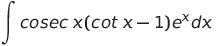Select Page

# 12 Science CBSE Maths Solutions for MCQ Probability in English

12 Science CBSE Maths Solutions for MCQ Probability in English to enable students to get Solutions in a narrative video format for the specific question.

Expert Teacher provides 12 Science CBSE Maths Solutions for MCQ Probability through Video Solutions in English language. This video solution will be useful for students to understand how to write an answer in exam in order to score more marks. This teacher uses a narrative style for a question from Probability not only to explain the proper method of answering question, but deriving right answer too.

Please find the question below and view the Solution in a narrative video format.

Question:

Solution Video in English:

You can select video Solutions from other languages also. Please check Solutions in ( Hindi )

## Similar Questions from CBSE, 12th Science, Maths, Probability

Question 1 : A bag contains 4 red and 4 black balls, another bag contains 2 red and 6 black balls. One of the two bags is selected at random and two balls are drawn at random (without replacement) from the bag which are both found to be red. Find the probability that the balls are drawn from the first bag.    (View Answer Video)

Question 2 : There are three coins. First is a biased that comes up tails 60% of the times, second is also a biased coin that comes up heads 75% of the times and third is an unbiased coin. One of the three coins is chosen at random and tossed, it shows heads, what is the probability that it was the first coin?      (View Answer Video)

Question 3 : Of the students in a college, it is known that 60% reside in hostel and 40% are day scholars (not residing in hostel). Previous year results report that 30% of all students who reside in hostel attain  'A' grade and 20% of day scholars attain 'A' grade in their annual examination. At the end of the year, one student is chosen at random from the college and he has an 'A' grade. What is the probability that the student is a hosteler?   (View Answer Video)

Question 4 : Ifandthen find P(E / F). (View Answer Video)

Question 5 : One card is drawn from a pack of 52 cards. Find the probability of getting a jack of hearts:

### Integrals

Question 1 : Evaluate :(View Answer Video)

Question 2 : Evaluate :(View Answer Video)

Question 3 : Evaluate:(View Answer Video)

Question 4 : Evaluate the following :(View Answer Video)

Question 5 : Find. (View Answer Video)

### Application of Derivatives

Question 1 : Ifthen the approximate value of f(3.02) is : (View Answer Video)

Question 2 : The function f is a differentiable function and satisfies the functional equation f(x) + f(y) = f(x + y) – xy – 1for every pair x, y of real numbers. If f(1) = 1, then the number of integers n ≠ 1 for which f(n) = n is_______________. (View Answer Video)

Question 3 : If, then the approximate value of f (3.02) is  (View Answer Video)

Question 4 : For all real values of x, the minimum value ofis  (View Answer Video)

Question 5 : The line y = mx + 1 is a tangent to the curveif the value of m is: (View Answer Video)

### Relations and Functions

Question 1 : Number of binary operations on the set { a, b} are: (View Answer Video)

Question 2 : Let A = {1, 2, 3}. Then, number of relations containing (1, 2) and (1, 3) which are reflexive and symmetric but not transitive is, (View Answer Video)

Question 3 : Functionsare defined respectively, by, find(View Answer Video)

Question 4 :  * is a binary operation on Z such that: a * b = a + b + ab. The solution of (3* 4) *x = – 1 is,